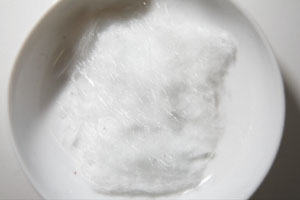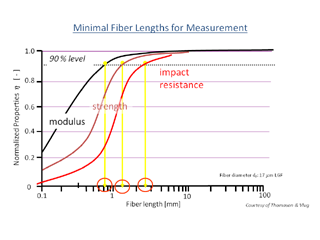# FASEP - Knowing the Fiber Length Distribution is Key

## FASEP Statistical Values

In addition to the number-averaged fiber length, i.e. the classic arithmetic mean of the fiber length (Ln), we also calculate the weight-average fiber length (Lp) (Lp or Ln are calculated according to the formulas of DIN ISO 22314), for the following reasons:• Ln is the average fiber length in the sample, but obscures the contribution of the long fibers.
• There are usually less long than short fibers in the component, but they represent a more significant weight fraction of the sample.
• Long fibers contribute more than short fibers to some mechanical properties. (Thomason and Vlug)
• Lp provides a more representative statement on the weighting of the fiber length in LFT materials, see following example: A sample with two 10mm fibers and one hundred 1mm fibers has a number average mean Fiber length of 1.1 mm, but the weight-average fiber length is 2.5 mm.

## Why is it important to know the fiber length percentage?Thomason and Vlug show the dependence of the various mechanical properties on the mean fiber length. The longer the mean fiber length, the higher the impact resistance, breaking strength and the modulus of elasticity of the material. This applies until a critical fiber length or a critical fiber content is reached.

## Advantages of fiber-reinforced plastics are:

• higher toughness
• higher fracture toughness
• less moisture sensitivity
• improved storage stability
• easier processing
• shorter cycle times in final production
• thermal postformability and weldability
• Recycling: can be melted again